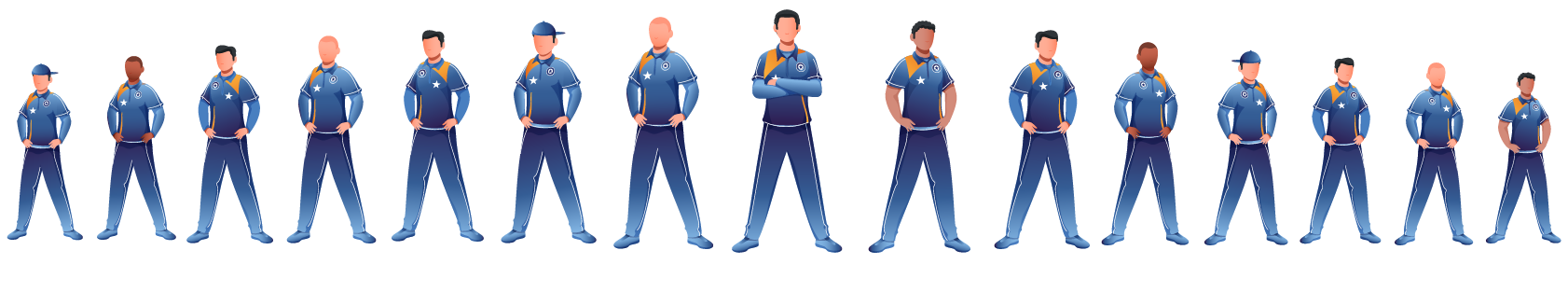# Combinations

Hey kids! Did you know that if you have a class of 15 students, then the total number of ways of making any 4 students out of 15, stand in a circle will be different than making them stand in a row? This is one of the properties of combinations. In this mini-lesson, we will be learning all combinations, along with permutations, solve examples on it and explore their applications in the real world.

For example, suppose we have a team of 15 football. We intend to select a playing team of 11 out of these 15 players. Thus, we want the number of ways in which we can select 11 players out of 15 players. (We are not interested in arranging those 11 players in a row - only the group/combination of those 11 players matters).## What is Meant by Combinations?

Combinations correspond to the selection of things (and not their arrangement). We do not intend to arrange things. We intend to select them.

Let us make this concept more specific. Suppose we have a set of 6 letters $$\left\{ {A,\,\,B,\,\,C,\,\,D,\,\,E,\,\,F} \right\}$$. In how many ways can we select a group of 3 letters from this set? Suppose we had to find the number of arrangements of 3 letters possible from those 6 letters. That number would be $${}^{\rm{6}}{P_3}$$  . Consider the permutations that contain the letters A, B and C. These are 3! = 6 in number, namely ABC, ACB, BAC, BCA, CAB and CBA.

Now, what we want is the number of combinations and not the number of arrangements. In other words, the 6 permutations listed above would correspond to a single combination. Differently put, the order of things is not important; only the group/combination matters. This means that the total number of combinations of 3 letters from the set of 6 letters available to us would be $${}^{\rm{6}}{P_3}/3!$$  since each combination is counted 3! times in the list of permutations. Thus, if we denote the number of combinations of 6 things taken 3 at a time by $${}^{\rm{6}}{C_3}$$, we have:

${}^{\rm{6}}{C_3} = \frac{{{}^{\rm{6}}{P_3}}}{{3!}}$

In general, suppose we have n things available to us, and we want to find the number of ways in which we can select r things out of these n things.

We first find the number of all the permutations of these n things taken r at a time. That number would be $${}^{\rm{n}}{P_r}$$  .  Now, in this list of $${}^{\rm{n}}{P_r}$$  permutations, each combination will be counted r! times since r things can be permuted amongst themselves in r! ways. Thus, the total number of permutations and combinations of these n things, taken r at a time, denoted by $${}^{\rm{n}}{C_r}$$, will be:

${}^n{C_r} = \frac{{{}^n{P_r}}}{{r!}} = \frac{{n!}}{{r!(n - r)!}}$

Example

Consider the word EDUCATION. This has 9 distinct letters. How many 3-letter permutations (words) can be formed using the letters of this word?We now know how to answer questions like this; the answer in this particular case will be $$^9{P_3}$$.

Consider the following 3-letter permutations formed using the letters A, E, T from EDUCATION:

AET    ATE

EAT    ETA

TAE    TEA

These 6 different arrangements correspond to the same selection of letters, which is {A, E, T}. Thus, in the list of all 3-letter permutations, we will find that each unique selection corresponds to 6 different arrangements. To find the number of unique 3-letter selections, we divide the number of 3-letter permutations by 6.

Hence, the number of 3-letter selections will be $$\frac{{^9{P_3}}}{6}$$.

## How to Calculate Combinations By Factorials?

Suppose that you have n different objects. You have to determine the number of unique r-selections (selections that contain r objects) which can be made from this group of n objects. Think of a group of n people – you have to find the number of unique sub-groups of size r, which can be created from this group.

The number of permutations of size r will be $$^n{P_r}$$. In the list of $$^n{P_r}$$ permutations, each unique selection will be counted $$r!$$ times, because the objects in an r-selection can be permuted amongst themselves in $$r!$$ ways. Thus, the number of unique selections will be $$\frac{{^n{P_r}}}{{r!}}$$.

$^n{C_r} = \frac{{^n{P_r}}}{{r!}} = \frac{{\left\{ {\frac{{n!}}{{\left( {n - r} \right)!}}} \right\}}}{{r!}} = \frac{{n!}}{{r!\left( {n - r} \right)!}}$

Examples

(i) Out of a group of 5 people, a pair needs to be formed. The number of ways in which this can be done is

$^5{C_2} = \frac{{5!}}{{2!\left( {5 - 2} \right)!}} = \frac{{5!}}{{2!3!}} = \frac{{120}}{{2 \times 6}} = 10$

(ii) The number of 4-letter selections which can be made from the letters of the word DRIVEN is

$^6{C_4} = \frac{{6!}}{{4!\left( {6 - 4} \right)!}} = \frac{{6!}}{{4!2!}} = \frac{{720}}{{24 \times 2}} = 15$

(iii) The number of ways of selecting 2 objects out of n is

\begin{align}&{}^nC_2=\frac{n!}{2!\left(n-2\right)!}=\frac{n\times\left(n-1\right)\times\left(n-2\right)!}{2\left(n-2\right)!}\\&\qquad\qquad\qquad\qquad=\frac{n\left(n-1\right)}2\end{align}

## What Is the General Formula of Combination?

A selection is also called a Combination. We denote the number of unique r-selections or combinations (out of a group of n objects) by $$^n{C_r}$$. Thus,

 $${}^n{C_r} = \frac{{{}^n{P_r}}}{{r!}} = \frac{{n!}}{{r!(n - r)!}}$$

Examples

The number of ways of selecting n objects out of n objects is:

$^n{C_n} = \frac{{n!}}{{n!\left( {n - n} \right)!}} = \frac{{n!}}{{n!0!}} = 1$

The number of ways of selecting 0 objects out of n objects is:

$^n{C_0} = \frac{{n!}}{{0!\left( {n - 0} \right)!}} = \frac{{n!}}{{0!n!}} = 1$

The number of ways of selecting 1 object out of n objects is:

$^n{C_1} = \frac{{n!}}{{1!\left( {n - 1} \right)!}} = \frac{{n \times \left( {n - 1} \right)!}}{{\left( {n - 1} \right)!}} = n$

Combinatorics
Combinatorics
Combinatorics
grade 9 | Questions Set 1
Combinatorics
grade 9 | Questions Set 2

More Important Topics
Numbers
Algebra
Geometry
Measurement
Money
Data
Trigonometry
Calculus